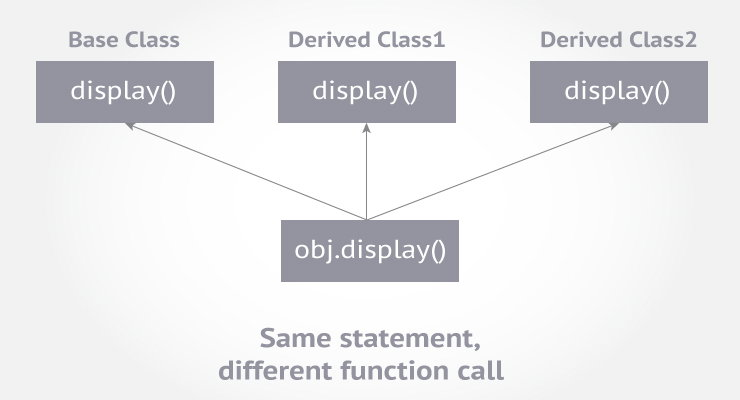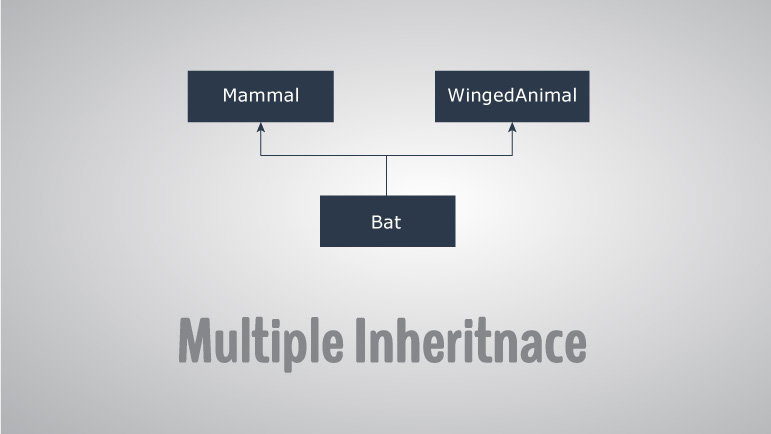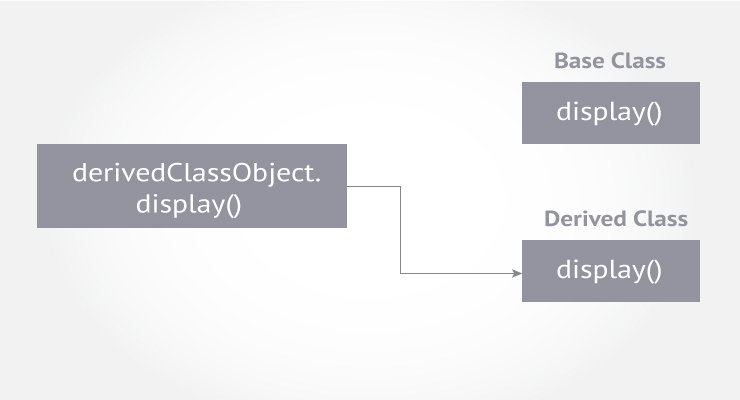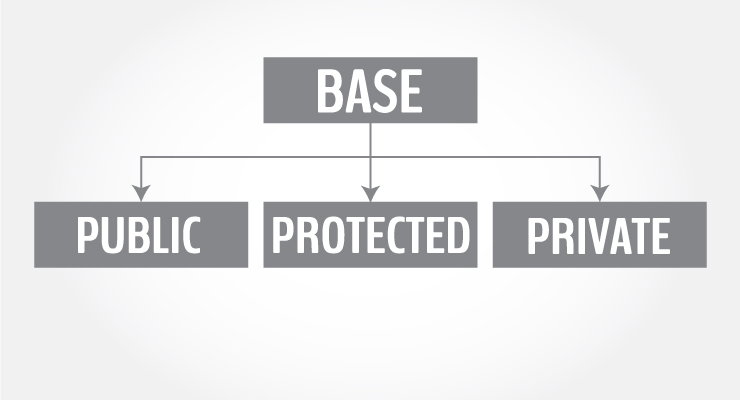# C++ Templates

In this article, you'll learn about templates in C++. You'll learn to use the power of templates for generic programming.

#Templates are powerful features of C++ which allows you to write generic programs. In simple terms, you can create a single function or a class to work with different data types using templates.
Templates are often used in larger codebase for the purpose of code reusability and flexibility of the programs.
The concept of templates can be used in two different ways:
• Function Templates
• Class Templates

## Function Templates

A function template works in a similar to a normal function, with one key difference.
A single function template can work with different data types at once but, a single normal function can only work with one set of data types.
Normally, if you need to perform identical operations on two or more types of data, you use function overloading to create two functions with the required function declaration.
However, a better approach would be to use function templates because you can perform the same task writing less and maintainable code.

### How to declare a function template?

A function template starts with the keyword template followed by template parameter/s inside  `< >` which is followed by function declaration.
```template <class T>
T someFunction(T arg)
{
... .. ...
}```
In the above code, T is a template argument that accepts different data types (int, float), and class is a keyword.
You can also use keyword `typename` instead of class in the above example.
When, an argument of a data type is passed to `someFunction( )`, compiler generates a new version of `someFunction()` for the given data type.

### Example 1: Function Template to find the largest number

Program to display largest among two numbers using function templates.
``````// If two characters are passed to function template, character with larger ASCII value is displayed.

#include <iostream>
using namespace std;

// template function
template <class T>
T Large(T n1, T n2)
{
return (n1 > n2) ? n1 : n2;
}

int main()
{
int i1, i2;
float f1, f2;
char c1, c2;

cout << "Enter two integers:\n";
cin >> i1 >> i2;
cout << Large(i1, i2) <<" is larger." << endl;

cout << "\nEnter two floating-point numbers:\n";
cin >> f1 >> f2;
cout << Large(f1, f2) <<" is larger." << endl;

cout << "\nEnter two characters:\n";
cin >> c1 >> c2;
cout << Large(c1, c2) << " has larger ASCII value.";

return 0;
}``````
Output
```Enter two integers:
5
10
10 is larger.

Enter two floating-point numbers:
12.4
10.2
12.4 is larger.

Enter two characters:
z
Z
z has larger ASCII value.
```

In the above program, a function template `Large()` is defined that accepts two arguments n1 and n2 of data type `T``T` signifies that argument can be of any data type.
`Large()` function returns the largest among the two arguments using a simple conditional operation.
Inside the `main()` function, variables of three different data types: `int``float` and `char`are declared. The variables are then passed to the `Large()` function template as normal functions.
During run-time, when an integer is passed to the template function, compiler knows it has to generate a `Large()` function to accept the int arguments and does so.
Similarly, when floating-point data and char data are passed, it knows the argument data types and generates the `Large()` function accordingly.
This way, using only a single function template replaced three identical normal functions and made your code maintainable.

### Example 2: Swap Data Using Function Templates

Program to swap data using function templates.
``````#include <iostream>
using namespace std;

template <typename T>
void Swap(T &n1, T &n2)
{
T temp;
temp = n1;
n1 = n2;
n2 = temp;
}

int main()
{
int i1 = 1, i2 = 2;
float f1 = 1.1, f2 = 2.2;
char c1 = 'a', c2 = 'b';

cout << "Before passing data to function template.\n";
cout << "i1 = " << i1 << "\ni2 = " << i2;
cout << "\nf1 = " << f1 << "\nf2 = " << f2;
cout << "\nc1 = " << c1 << "\nc2 = " << c2;

Swap(i1, i2);
Swap(f1, f2);
Swap(c1, c2);

cout << "\n\nAfter passing data to function template.\n";
cout << "i1 = " << i1 << "\ni2 = " << i2;
cout << "\nf1 = " << f1 << "\nf2 = " << f2;
cout << "\nc1 = " << c1 << "\nc2 = " << c2;

return 0;
}``````
Output
```Before passing data to function template.
i1 = 1
i2 = 2
f1 = 1.1
f2 = 2.2
c1 = a
c2 = b

After passing data to function template.
i1 = 2
i2 = 1
f1 = 2.2
f2 = 1.1
c1 = b
c2 = a
```
In this program, instead of calling a function by passing a value, a call by reference is issued.
The `Swap()` function template takes two arguments and swaps them by reference.

## Class Templates

Like function templates, you can also create class templates for generic class operations.
Sometimes, you need a class implementation that is same for all classes, only the data types used are different.
Normally, you would need to create a different class for each data type OR create different member variables and functions within a single class.
This will unnecessarily bloat your code base and will be hard to maintain, as a change is one class/function should be performed on all classes/functions.
However, class templates make it easy to reuse the same code for all data types.

### How to declare a class template?

```template <class T>
class className
{
... .. ...
public:
T var;
T someOperation(T arg);
... .. ...
};```
In the above declaration, `T` is the template argument which is a placeholder for the data type used.
Inside the class body, a member variable var and a member function `someOperation()` are both of type `T`.

### How to create a class template object?

To create a class template object, you need to define the data type inside a `< >` when creation.
```className<dataType> classObject;
```
For example:
```className<int> classObject;
className<float> classObject;
className<string> classObject;
```

### Example 3: Simple calculator using Class template

Program to add, subtract, multiply and divide two numbers using class template
``````#include <iostream>
using namespace std;

template <class T>
class Calculator
{
private:
T num1, num2;

public:
Calculator(T n1, T n2)
{
num1 = n1;
num2 = n2;
}

void displayResult()
{
cout << "Numbers are: " << num1 << " and " << num2 << "." << endl;
cout << "Subtraction is: " << subtract() << endl;
cout << "Product is: " << multiply() << endl;
cout << "Division is: " << divide() << endl;
}

T add() { return num1 + num2; }

T subtract() { return num1 - num2; }

T multiply() { return num1 * num2; }

T divide() { return num1 / num2; }
};

int main()
{
Calculator<int> intCalc(2, 1);
Calculator<float> floatCalc(2.4, 1.2);

cout << "Int results:" << endl;
intCalc.displayResult();

cout << endl << "Float results:" << endl;
floatCalc.displayResult();

return 0;
}``````
Output
```Int results:
Numbers are: 2 and 1.
Subtraction is: 1
Product is: 2
Division is: 2

Float results:
Numbers are: 2.4 and 1.2.
Subtraction is: 1.2
Product is: 2.88
Division is: 2

```
In the above program, a class template `Calculator` is declared.
The class contains two private members of type `T`num1 & num2, and a constructor to initalize the members.
It also contains public member functions to calculate the addition, subtraction, multiplication and division of the numbers which return the value of data type defined by the user. Likewise, a function `displayResult()` to display the final output to the screen.
In the `main()` function, two different `Calculator` objects `intCalc` and `floatCalc` are created for data types: `int` and `float` respectively. The values are initialized using the constructor.
Notice we use `<int>` and `<float>` while creating the objects. These tell the compiler the data type used for the class creation.
This creates a class definition each for `int` and `float`, which are then used accordingly.
Then, `displayResult()` of both objects is called which performs the Calculator operations and displays the output

# C++ Virtual Function

In this article, you will learn about virtual function and where to use it. Also, you will learn about pure virtual function and abstract class.

#A virtual function is a member function in base class that you expect to redefine in derived classes.
Before going into detail, let's build an intuition on why virtual functions are needed in the first place.

## An Example to Begin With

Let us assume, we are working on a game (weapons specifically).
We created the Weapon class and derived two classes Bomb and Gun to load features of respective weapons.
``````#include <iostream>
using namespace std;

class Weapon
{
public:
};

class Bomb : public Weapon
{
public:
};

class Gun : public Weapon
{
public:
};

int main()
{
Weapon *w = new Weapon;
Bomb *b = new Bomb;
Gun *g = new Gun;

return 0;
}``````
Output
```Loading weapon features.
We defined three pointer objects wb and g of classes WeaponBomb and Gunrespectively. And, we called `loadFeatures()` member function of each objects using:
```w->loadFeatures();
Works perfectly!
However, our game project started getting bigger and bigger. And, we decided to create a separate Loader class to load weapon features.
``````
{
public:
{
weapon->features();
}
};``````
The `loadFeatures()` loads the feature of a specific weapon.

### Let's try to implement our Loader class

``````#include <iostream>
using namespace std;

class Weapon
{
public:
``` Weapon() { cout << "Loading weapon features.\n"; }```

void features()
};

class Bomb : public Weapon
{
public:
void features()
{
```this->Weapon::features();` ` ```            cout << "Loading bomb features.\n";
}
};

class Gun : public Weapon
{
public:
void features()
{
```this->Weapon::features();```
}
};

{
public:
{
weapon->features();
}
};

int main()
{
Weapon *w;
Bomb b;
Gun g;

w = &b;

w = &g;

return 0;
}``````
Output
```Loading weapon features.
```
Our implementation seemed correct. However, weapon features was loaded 4 times. Why?
Initially, the Weapon object w is pointing to the b object (of Bomb) class. And, we tried to load the features of Bomb object by passing it to `loadFeatures()` function using l object to pointer (of Loader class).
Similarly, we tried to load the features of Gun object.
However, the `loadFeatures()` function of the Loader class takes pointer to object of a Weapon class as an argument:
`void loadFeatures(Weapon *weapon)`
That's the reason weapon features are loaded 4 times. To solve this issue, we need to make function of base class (Weapon class) virtual using virtual keyword.
``````class Weapon
{
public:
virtual void features()
};``````

## Example: Using Virtual Function to Solve the Problem

``````#include <iostream>
using namespace std;

class Weapon
{
public:
virtual void features()
};

class Bomb : public Weapon
{
public:
void features()
{ ```this->Weapon::features();   ```cout << "Loading bomb features.\n";
}
};

class Gun : public Weapon
{
public:
void features()
{
```this->Weapon::features(); ```            cout << "Loading gun features.\n";
}
};

{
public:
{
weapon->features();
}
};

int main()
{
Weapon *w;
Bomb b;
Gun g;

w = &b;

w = &g;

return 0;
}``````
Output
```Loading weapon features.
```
Also, notice that, the `l->loadFeatures(w)` function calls the function of different classes depending upon what l object is pointing.
Using virtual function made our code not only clearer but flexible too.
In the above program, weapon features is printed twice. We encourage you to add additional code on the above program to load weapon features only once.
If we want to add another weapon (let's say knife), we can easily add and load features of it. How?
``````class Knife : public Weapon
{
public:
void features()
{
```this->Weapon::features(); ```            cout << "Loading knife features.\n";
}
};``````
And, in `main()` function.
```Knife k;
w = &k;
It's worth noticing that we didn't change anything in the Loader class to load features of knife.

## C++ Abstract class and Pure virtual Function

The goal of object-oriented programming is to divide a complex problem into small sets. This helps understand and work with problem in an efficient way.
Sometimes, it's desirable to use inheritance just for the case of better visualization of the problem.
In C++, you can create an abstract class that cannot be instantiated (you cannot create object of that class). However, you can derive a class from it and instantiate object of the derived class.
Abstract classes are the base class which cannot be instantiated.
A class containing pure virtual function is known as abstract class.

## Pure Virtual Function

A virtual function whose declaration ends with =0 is called a pure virtual function. For example,
```class Weapon
{
public:
virtual void features() = 0;
};
```
Here, the pure virtual function is
`virtual void features() = 0`
And, the class Weapon is an abstract class.

## Example: Abstract Class and Pure Virtual Function

``````#include <iostream>
using namespace std;

// Abstract class
class Shape
{
protected:
float l;
public:
void getData()
{
cin >> l;
}

// virtual Function
virtual float calculateArea() = 0;
};

class Square : public Shape
{
public:
float calculateArea()
{   return l*l;  }
};

class Circle : public Shape
{
public:
float calculateArea()
{ return 3.14*l*l; }
};

int main()
{
Square s;
Circle c;

cout << "Enter length to calculate the area of a square: ";
s.getData();
cout<<"Area of square: " << s.calculateArea();
cout<<"\nEnter radius to calculate the area of a circle: ";
c.getData();
cout << "Area of circle: " << c.calculateArea();

return 0;
}``````
Output
```Enter length to calculate the area of a square: 4
Area of square: 16
Enter radius to calculate the area of a circle: 5
Area of circle: 78.5```
In this program, pure virtual function `virtual float area() = 0;` is defined inside the Shape class.
One important thing to note is that, you should override the pure virtual function of the base class in the derived class. If you fail the override it, the derived class will become an abstract class as well

# C++ friend Function and friend Classes

In this article, you'll learn to create friend function and class in C++, and use them efficiently in your program.

#One of the important concepts of OOP is data hiding, i.e., a nonmember function cannot access an object's private or protected data.
But, sometimes this restriction may force programmer to write long and complex codes. So, there is mechanism built in C++ programming to access private or protected data from non-member functions.
This is done using a friend function or/and a friend class.

## friend Function in C++

If a function is defined as a friend function then, the private and protected data of a class can be accessed using the function.
The complier knows a given function is a friend function by the use of the keyword friend.
For accessing the data, the declaration of a friend function should be made inside the body of the class (can be anywhere inside class either in private or public section) starting with keyword `friend`.

### Declaration of friend function in C++

```class class_name
{
... .. ...
friend return_type function_name(argument/s);
... .. ...
}
```
Now, you can define the friend function as a normal function to access the data of the class. No `friend` keyword is used in the definition.
```class className
{
... .. ...
friend return_type functionName(argument/s);
... .. ...
}

return_type functionName(argument/s)
{
... .. ...
// Private and protected data of className can be accessed from
// this function because it is a friend function of className.
... .. ...
}
```

### Example 1: Working of friend Function

``````/* C++ program to demonstrate the working of friend function.*/
#include <iostream>
using namespace std;

class Distance
{
private:
int meter;
public:
Distance(): meter(0) { }
//friend function
};

// friend function definition
{
//accessing private data from non-member function
d.meter += 5;
return d.meter;
}

int main()
{
Distance D;
return 0;
}``````

Output
`Distance: 5`
Here, friend function `addFive()` is declared inside `Distance` class. So, the private data meter can be accessed from this function.
Though this example gives you an idea about the concept of a friend function, it doesn't show any meaningful use.
A more meaningful use would to when you need to operate on objects of two different classes. That's when the friend function can be very helpful.
You can definitely operate on two objects of different classes without using the friend function but the program will be long, complex and hard to understand.

### Example 2: Addition of members of two different classes using friend Function

``````#include <iostream>
using namespace std;

// forward declaration
class B;
class A {
private:
int numA;
public:
A(): numA(12) { }
// friend function declaration
};

class B {
private:
int numB;
public:
B(): numB(1) { }
// friend function declaration
};

// Function add() is the friend function of classes A and B
// that accesses the member variables numA and numB
{
return (objectA.numA + objectB.numB);
}

int main()
{
A objectA;
B objectB;
return 0;
}``````
Output
`Sum: 13`
In this program, classes A and B have declared `add()` as a friend function. Thus, this function can access private data of both class.
Here, `add()` function adds the private data numA and numB of two objects objectA and objectB, and returns it to the main function.
To make this program work properly, a forward declaration of a class `class B` should be made as shown in the above example.
This is because `class B` is referenced within the `class A` using code: `friend int add(A , B);`.

## friend Class in C++ Programming

Similarly, like a friend function, a class can also be made a friend of another class using keyword `friend`. For example:
```... .. ...
class B;
class A
{
// class B is a friend class of class A
friend class B;
... .. ...
}

class B
{
... .. ...
}
```
When a class is made a friend class, all the member functions of that class becomes friend functions.
In this program, all member functions of `class B` will be friend functions of `class A`. Thus, any member function of class B can access the private and protected data of class A. But, member functions of class A cannot access the data of class B.
Remember, friend relation in C++ is only granted, not taken

# C++ Multiple, Multilevel and Hierarchical Inheritance

In this article, you will learn about different models of inheritance in C++ programming: Multiple, Multilevel and Hierarchical inheritance with examples.

#Inheritance is one of the core feature of an object-oriented programming language. It allows software developers to derive a new class from the existing class. The derived class inherits the features of the base class (existing class).
There are various models of inheritance in C++ programming.

## C++ Multilevel Inheritance

In C++ programming, not only you can derive a class from the base class but you can also derive a class from the derived class. This form of inheritance is known as multilevel inheritance.
```class A
{
... .. ...
};
class B: public A
{
... .. ...
};
class C: public B
{
... ... ...
};```
Here, class B is derived from the base class A and the class C is derived from the derived class B.

## Example 1: C++ Multilevel Inheritance

``````#include <iostream>
using namespace std;

class A
{
public:
void display()
{
cout<<"Base class content.";
}
};

class B : public A
{

};

class C : public B
{

};

int main()
{
C obj;
obj.display();
return 0;
}``````

Output
`Base class content.`
In this program, class C is derived from class B (which is derived from base class A).
The obj object of class C is defined in the `main()` function.
When the `display()` function is called, `display()` in class A is executed. It's because there is no `display()` function in class C and class B.
The compiler first looks for the `display()` function in class C. Since the function doesn't exist there, it looks for the function in class B (as C is derived from B).
The function also doesn't exist in class B, so the compiler looks for it in class A (as B is derived from A).
If `display()` function exists in C, the compiler overrides `display()` of class A (because of member function overriding).

## C++ Multiple Inheritance

In C++ programming, a class can be derived from more than one parents. For example: A class Bat is derived from base classes Mammal and WingedAnimal. It makes sense because bat is a mammal as well as a winged animal.## Example 2: Multiple Inheritance in C++ Programming

``````#include <iostream>
using namespace std;

class Mammal {
public:
Mammal()
{
cout << "Mammals can give direct birth." << endl;
}
};

class WingedAnimal {
public:
WingedAnimal()
{
cout << "Winged animal can flap." << endl;
}
};

class Bat: public Mammal, public WingedAnimal {

};

int main()
{
Bat b1;
return 0;
}``````
Output
```Mammals can give direct birth.
Winged animal can flap.```

### Ambiguity in Multiple Inheritance

The most obvious problem with multiple inheritance occurs during function overriding.
Suppose, two base classes have a same function which is not overridden in derived class.
If you try to call the function using the object of the derived class, compiler shows error. It's because compiler doesn't know which function to call. For example,
``````class base1
{
public:
void someFunction( )
{ .... ... .... }
};
class base2
{
void someFunction( )
{ .... ... .... }
};
class derived : public base1, public base2
{

};

int main()
{
derived obj;

obj.someFunction() // Error!
}
``````
This problem can be solved using scope resolution function to specify which function to class either base1 or base2
```int main()
{
obj.base1::someFunction( );  // Function of base1 class is called
obj.base2::someFunction();   // Function of base2 class is called.
}```

## C++ Hierarchical Inheritance

If more than one class is inherited from the base class, it's known as hierarchical inheritance. In hierarchical inheritance, all features that are common in child classes are included in the base class.
For example: Physics, Chemistry, Biology are derived from Science class.

### Syntax of Hierarchical Inheritance

```class base_class {
... .. ...
}

class first_derived_class: public base_class {
... .. ...
}

class second_derived_class: public base_class {
... .. ...
}

class third_derived_class: public base_class {
... .. ...
}```

# C++ Function Overriding

In this article, you will learn about function overriding. Also, you will learn how can assess the overridden function of the base class in C++ programming.# Public, Protected and Private Inheritance in C++ Programming

In this article, you'll learn to use public, protected and private inheritance in C++. You'll learn where and how it is used, with examples.

#You can declare a derived class from a base class with different access control, i.e., public inheritance, protected inheritance or private inheritance.
```#include <iostream>
using namespace std;

class base
{
.... ... ....
};

class derived : access_specifier base
{
.... ... ....
};
```
Note: Either publicprotected or private keyword is used in place of access_specifierterm used in the above code.

## Example of public, protected and private inheritance in C++

``````class base
{
public:
int x;
protected:
int y;
private:
int z;
};

class publicDerived: public base
{
// x is public
// y is protected
// z is not accessible from publicDerived
};

class protectedDerived: protected base
{
// x is protected
// y is protected
// z is not accessible from protectedDerived
};

class privateDerived: private base
{
// x is private
// y is private
// z is not accessible from privateDerived
}``````
In the above example, we observe the following things:
• `base` has three member variables: x, y and z which are `public``protected` and `private` member respectively.
• `publicDerived` inherits variables x and y as public and protected. z is not inherited as it is a private member variable of base.
• `protectedDerived` inherits variables x and y. Both variables become protected. z is not inherited
If we derive a class `derivedFromProtectedDerived` from protectedDerived, variables x and y are also inherited to the derived class.
• `privateDerived` inherits variables x and y. Both variables become private. z is not inherited
If we derive a class `derivedFromPrivateDerived` from privateDerived, variables x and y are not inherited because they are private variables of privateDerived.

### Accessibility in Public Inheritance

Accessibility private variables protected variables public variables
Accessible from own class? yes yes yes
Accessible from derived class? no yes yes
Accessible from 2nd derived class? no yes yes

### Accessibility in Protected Inheritance

Accessibility private variables protected variables public variables
Accessible from own class? yes yes yes
Accessible from derived class? no yes yes
(inherited as protected variables)
Accessible from 2nd derived class? no yes yes

### Accessibility in Private Inheritance

Accessibility private variables protected variables public variables
Accessible from own class? yes yes yes
Accessible from derived class? no yes
(inherited as private variables)
yes
(inherited as private variables)
Accessible from 2nd derived class? no no no